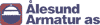Metric System 08-000-007
UNDERSTANDING THE METRIC SYSTEM
The Metric System is based on powers of ten. The table below shows the progression of prefixes.
 PREFIX SYMBOL MATHEMATICAL VALUE exa- E 1,000,000,000,000,000,000 peta- P 1,000,000,000,000,000 tera- T 1,000,000,000,000 giga- G 1,000,000,000 meg- M 1,000,000 kilo- k 1,000 hecto- h 100 deka- da 10 (meter, liter, gram) 1 deci- d 0.1 centi- c 0.01 milli- m 0.001 micro- µ 0.000,001 nano- n 0.000,000,001 pico- p 0.000,000,000,001 femto- f 0.000,000,000,000,001 atto- a 0.000,000,000,000,000,001

The unit of measurement from length is the meter. The progression of the
more common units of measure are:

 kilometer km 1,000 meters meter m 1.0 centimeter cm 0.01 meters millimeter mm 0.001 meters nanometers nm 0.000,000,001 meters

The unit of measure for capacity is the liter, so the measurements allow
the same progression, such as kiloliter, centiliter, and so on.

The unit of measure for weight is the gram, so the measurement prefixes
are kilogram, centigram, and so forth.

When making conversions within the metric system, each change in prefix
represents one decimal point move. If you move downward one the chart,
the decimal places will be added. If you move upward, the decimal places
are moved to the left.

 13.12.2005